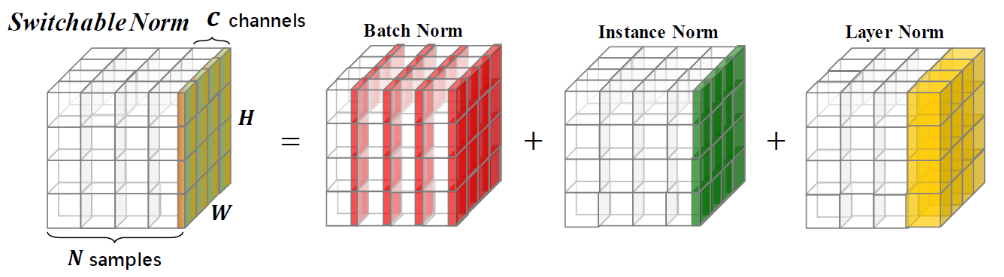# Learning-to-Learn-to-Normalize: Algorithms, Applications and TheoryDate
Dec 18, 2018 4:37 PM

We present a new family of normalization methods in Deep Learning, opening up new research directions in applications, algorithms, and theory of deep neural networks. Various deep models and tasks could be revisited by these techniques. They facilitate theoretical understanding of deep neural networks.

GWNN1: The Generalized Whitened Neural Networks (GWNNs) draws a connection between forward computations and backward computations of a DNN. Training a GWNN by using SGD mimics the behavior of NGD. It shows when $\mathrm{SGD}\approx\mathrm{NGD}$ by only changing the network architectures, that is, whitening the input of hidden layer.

EigenNet2: EigenNet was a generalization of GWNN by whitening both the forward hidden features and backward gradients of a DNN, making the Fisher Information Matrix well-conditioned and training an EigenNet by using SGD mimics the behavior of NGD.

Kalman Normalization3: GWNN and EigenNet estimated the covariance matrices by assuming layers are independent. Kalman Normalization (KN) was the first work that explores relationships between hidden layers to normalize the hidden features, where the estimated $\mu^\ell$ and $\Sigma^\ell$ are propagated through the deep network by learning a transition matrix $E$.

Switch Whitening (SW): SW is able to switch between standardization and whitening to reduce computations.

IBN-Net4: IBN-Net carefully unifies instance normalization and batch normalization in a single deep network. It provides a simple way to increase both modeling and generalization capacity without increasing computational complexity.

Switchable Normalization (SN)5: SN was the first meta normalization algorithm that is easily trained in a differentiable way. SN learns to combine BN, IN, LN, and GN for each hidden layer. It provides a new point of view for the deep learning community. SN also has theoretical value because analyzing each normalization method in a unified framework is necessary before analyzing their interactions in SN. Understanding existing normalization approaches are still open problems, not to mention their interactions in optimization and generalization. Moreover, a unified formulation of normalization approaches is still elusive.Switchable Normalization (SN) learns to normalize different layers by using different normalization methods.

Synchronized SN: Sync SN synchronizes BN in SN across multiple machines and GPUs. It typically performs better than SN and existing normalizers.

Understanding SN6: SN offers a new perspective in deep learning. We believe many DNNs could be reexamined with this new perspective, which therefore deserves thorough analyses. Unlike the original SN paper only visualized ratios when training converged, we study the learning dynamics and generalization regarding various hyper-parameters. We found that using hard ratios in SN improves soft ratios in SN by $0.5$ top-1 classification accuracy in ImageNet, that is $76.9~v.s. 77.4$.

Sparse SN (SSN)7: SN uses softmax function to learn importance ratios to combine normalizers, leading to redundant computations compared to a single normalizer. The importance ratios of SSN are constrained to be sparse. Unlike $\ell_1$ and $\ell_0$ constraints that impose difficulties in optimization, SNN turns this sparse constrained optimization problem into feed-forward computation by proposing SparsestMax, which is a sparse version of softmax. SSN inherits all benefits from SN such as applicability in various tasks and robustness to a wide range of batch sizes. SSN is guaranteed to select only one normalizer for each normalization layer, avoiding redundant computations.

Regularization in BN8: We are trying to understand different normalizers theoretically, for example, batch normalization. This work understands convergence and generalization of BN theoretically. We analyze BN by using a basic block of neural networks, consisting of a kernel layer, a BN layer, and a nonlinear activation function. This basic network helps us understand the impacts of BN in three aspects. By viewing BN as an implicit regularizer, BN can be decomposed into population normalization (PN) and gamma decay as an explicit regularization. Learning dynamics of BN and the regularization show that training converged with large maximum and effective learning rate. Generalization of BN is explored by using statistical mechanics.

Meta Normalization (MN)9: MN provides a unified formulation for all normalization methods. MN is able to learn arbitrary normalization operations for different convolutional layers in a deep ConvNet. Unlike existing approaches that manually defined the statistics (mean and variance), MN learns to estimate them. MN adapts to various networks, tasks, and batch sizes. MN can be easily implemented and trained in a differentiable end-to-end manner with merely small number of parameters. MN’s matrix formulation represents a wide range of normalization methods, shedding light on analyzing them holistically.

1. Ping Luo, Learning Deep Architectures via Generalized Whitened Neural Networks, ICML 2017 ↩︎

2. Ping Luo, EigenNet: Towards Fast and Structural Learning of Deep Neural Networks, IJCAI 2017 ↩︎

3. Guangrun Wang, Ping Luo, Xinjiang Wang, Liang Lin, Kalman Normalization: Normalizing Internal Representations Across Network Layers, NeuralPS 2018 ↩︎

4. Xingang Pan, Ping Luo, Jianping Shi, Xiaoou Tang, Two at Once: Enhancing Learning and Generalization Capacities via IBN-Net, ECCV 2018 ↩︎

5. Ping Luo, Jiamin Ren, Zhanglin Peng, Ruimao Zhang, Jingyu Li, Differentiable Learning-to-Normalize via Switchable Normalization, ICLR 2019 ↩︎

6. Ping Luo, Zhanglin Peng, Jiamin Ren, Ruimao Zhang, Do Normalization Layers in a Deep ConvNet Really Need to Be Distinct?, arXiv:1811.07727, 2019 ↩︎

7. Wenqi Shao, Tianjian Meng, Jingyu Li, Ruimao Zhang, Yudian Li, Xiaogang Wang, Ping Luo, SSN: Learning Sparse Switchable Normalization via SparsestMax, CVPR 2019 ↩︎

8. Ping Luo, Xinjiang Wang, Wenqi Shao, Zhanglin Peng, Towards Understanding Regularization in Batch Normalization, ICLR 2019 ↩︎

9. Ping Luo, Zhanglin Peng, Wenqi Shao, Ruimao Zhang, Jiamin Ren, Wu lingyun, Differentiable Learning to Learn to Normalize, in submission, 2019 ↩︎